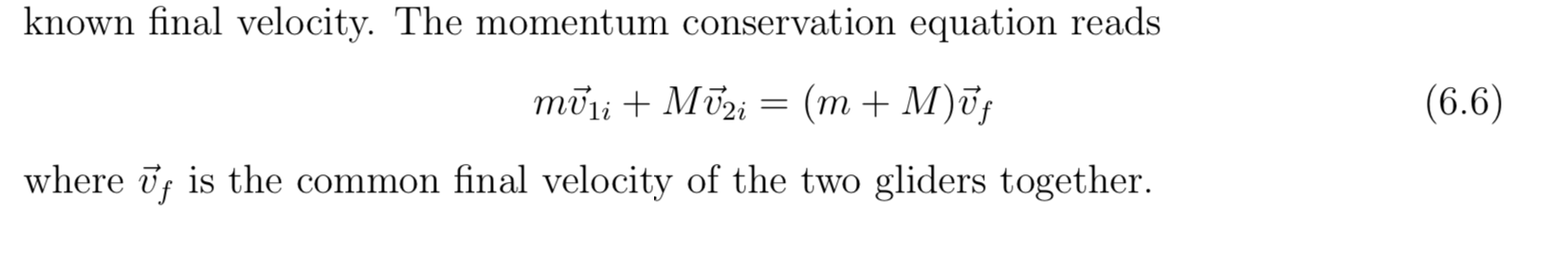# known final velocity. The momentum conservation equation reads(m + M)ūj(6.6)moliMiwhere f is the common final velocity of the two gliders together

Question
21 views

Inelastic Collision

Calculate the combined velocity of the two gliders after collision collision from the v1i and v2i from values below using the attached equation.

M1 : 195.2g ± 0.1g

M2: 182.8g ± 0.1g

 M1 (needle) M2 (wax) Intial Velocity 0.28m/s Intial Velocity 0.0m/s Final Velocity 0.15m/s Final Velocity 0.15m/shelp_outlineImage Transcriptioncloseknown final velocity. The momentum conservation equation reads (m + M)ūj (6.6) moliMi where f is the common final velocity of the two gliders together fullscreen
check_circle

Step 1

According to the conservation of momentum equation,

Step 2

Substitute the values, to calculate the combined v...

### Want to see the full answer?

See Solution

#### Want to see this answer and more?

Solutions are written by subject experts who are available 24/7. Questions are typically answered within 1 hour.*

See Solution
*Response times may vary by subject and question.
Tagged in

### Kinematics